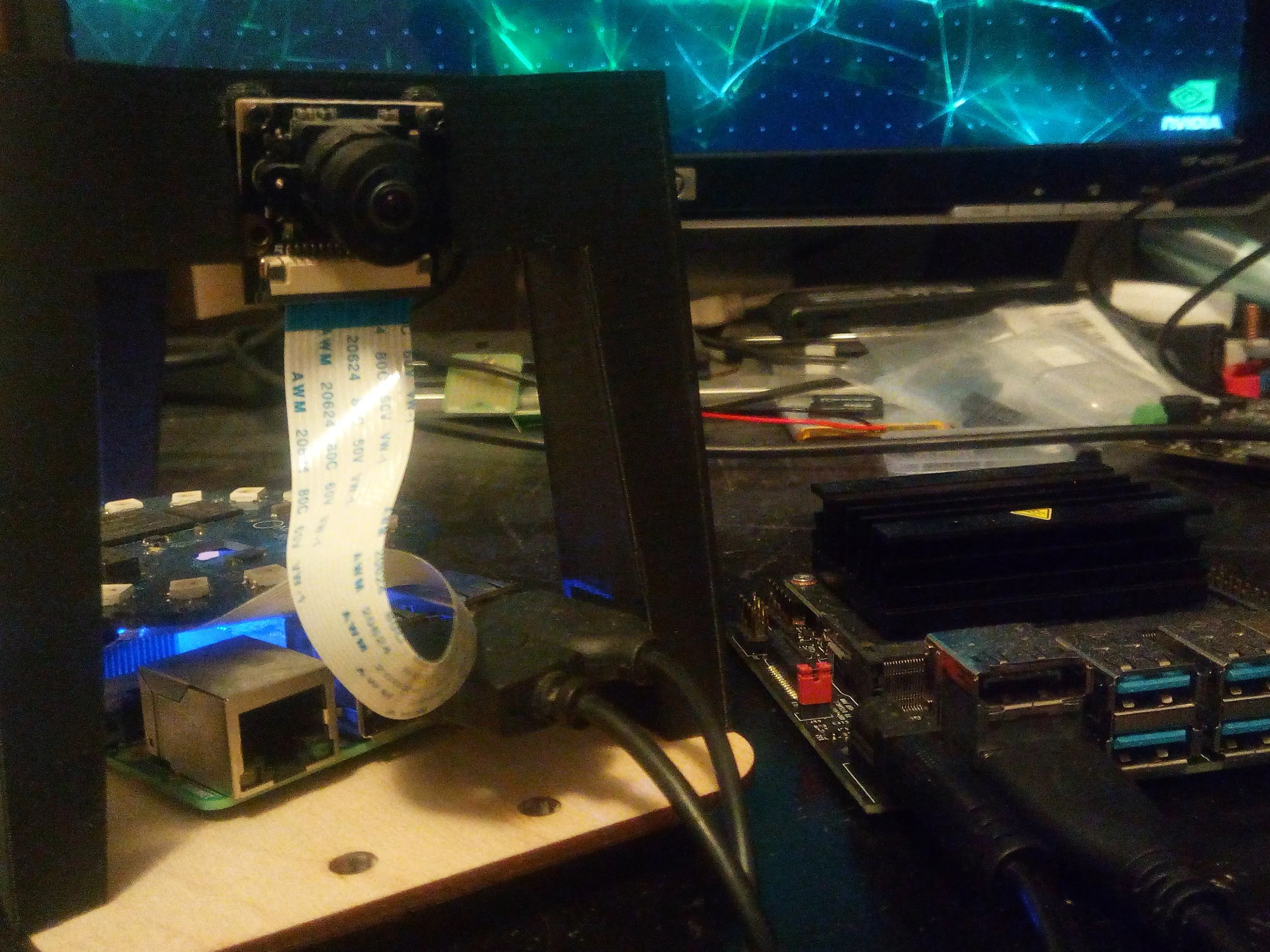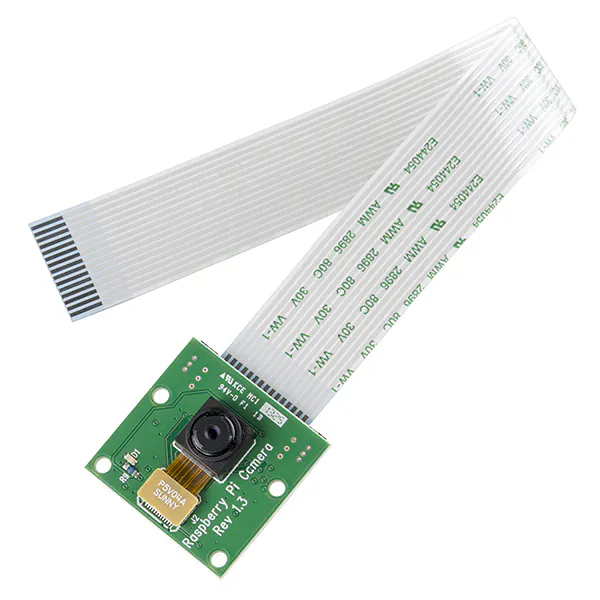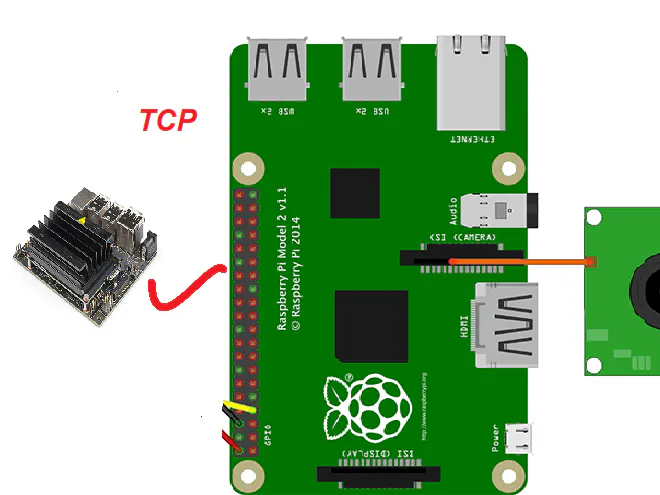# Voice Controlled AI Camera

Build a voice controlled AI camera that notifies with what it sees via email.## Things used in this project

### Hardware componentsRaspberry Pi 3 Model B
×1NVIDIA Jetson Nano Developer Kit
×1Raspberry Pi Camera Module
×1

### Software apps and online servicesTensorFlowOpenCV

## Schematics

### AI camera system conceptual block diagram

AI camera system conceptual block diagram## Code

### TestSendEmailImage.py

Python
Tests sending images via email.
```# Copyright dhq November 13 2019

# import all necessary components
import smtplib
from email.mime.text import MIMEText
from email.mime.image import MIMEImage
from email.mime.multipart import MIMEMultipart
import pickle
import socket
import struct
from PIL import Image
import numpy as np
import time
import os, sys
import sys
import cv2
import smtplib
import numpy as np

from imutils.video import VideoStream
import datetime
import argparse
import imutils
import time
import cv2
from time import sleep

HOSTNAME = 'smtp-mail.outlook.com'
SUBJECT = 'Subject: AI camera using (imageNetV3) engine detected: '

""" Get email credentials
:param none:
:returns none :
"""

EMAIL_BODY = SUBJECT + str(detectedobject)

msg = MIMEMultipart('related')
msg['Subject'] = SUBJECT

html = """\
<html>
<body>
<img src="cid:image1" alt="Logo" style="width:300px;height:400px;"><br>
<p><h4 style="font-size:15px;">AI engine camera snapshot.</h4></p>
</body>
</html>
"""
# Record the MIME types of text/html.
part2 = MIMEText(html, 'html')

# Attach parts into message container.
msg.attach(part2)

# This example assumes the image is in the current directory
fp = open('ELE.JPG', 'rb')
fp.close()

msg.attach(msgImage)

# print(msg.as_string())
server = smtplib.SMTP(HOSTNAME, 587)
server.ehlo()
server.starttls()
server.quit()
print ("Email sent. Logging Out...")

if __name__ == '__main__':
try:
print ('To exit the program press Ctrl-C.')
detectedobject = str("found another lama")
print ('Sending email \r\n.')
print ('Email sent \r\n.')

except KeyboardInterrupt:
print ('Quitting...')

```

### ImgServerAI.py

Python
```# Copyright dhq November 13 2019

# import all necessary components
import smtplib
from email.mime.text import MIMEText
from email.mime.image import MIMEImage
from email.mime.multipart import MIMEMultipart

import socket
import sys
import cv2
import pickle
import numpy as np
import struct ## new
import zlib

from PIL import Image
import os
import sys

# from skimage.transform import resize
# import numpy as np
# from matplotlib import pyplot as plt
# from skimage import data, io
# from matplotlib import pyplot as plt
# from skimage import img_as_ubyte		#convert float to uint8
# from skimage.color import rgb2gray

from tensorflow.python.keras.preprocessing import image
from tensorflow.python.keras.applications.inception_v3 import *
import numpy as np

from imutils.video import VideoStream
import datetime
import argparse
import imutils
import time
import cv2
from time import sleep

HOST=''
PORT=8485

HOSTNAME = 'smtp-mail.outlook.com'
SUBJECT = 'Subject: AI camera using (imageNetV3) engine detected: '

""" Get email credentials
:param none:
:returns none :
"""

EMAIL_BODY = SUBJECT + str(detectedobject)

msg = MIMEMultipart('related')
msg['Subject'] = SUBJECT

html = """\
<html>
<body>
<img src="cid:image1" alt="Logo" style="width:300px;height:400px;"><br>
<p><h4 style="font-size:15px;">AI engine camera snapshot.</h4></p>
</body>
</html>
"""
# Record the MIME types of text/html.
part2 = MIMEText(html, 'html')

# Attach parts into message container.
msg.attach(part2)

# This example assumes the image is in the current directory
fp = open('servpic.jpg', 'rb')
fp.close()

msg.attach(msgImage)

# print(msg.as_string())
server = smtplib.SMTP(HOSTNAME, 587)
server.ehlo()
server.starttls()
server.quit()
print ("Email sent. ")

def main():
model = InceptionV3(weights='imagenet')
print('Deep CNN started')

s=socket.socket(socket.AF_INET,socket.SOCK_STREAM)
print('Socket created')

s.bind((HOST,PORT))
print('Socket bind complete')
s.listen(10)
print('Socket now listening')

data = b""
while True:
print("Recv: {}".format(len(data)))
data += conn.recv(4096)

print("Done Recv: {}".format(len(data)))
msg_size = struct.unpack(">L", packed_msg_size)
print("msg_size: {}".format(msg_size))
while len(data) < msg_size:
data += conn.recv(4096)
frame_data = data[:msg_size]
data = data[msg_size:]
print(msg_size)
cv2.imwrite("servpic.jpg",frame)
# cv2.imshow('ImageWindow',frame)
# cv2.waitKey(1)
x = image.img_to_array(img)
x = np.expand_dims(x, axis=0)
x = preprocess_input(x)
y = model.predict(x)
# cnt =  cnt + 1
for index, res in enumerate(decode_predictions(y)):
print('{}. {}: {:.3f}%'.format(index + 1, res, 100 * res))
print(decode_predictions(y))				#this is a list
print(type(decode_predictions(y)))	#this is a tuple
print("Item detected : " + (decode_predictions(y)))		# this picks the class description from the first tuple that shows the class with the highest probabililty
decobj = (decode_predictions(y))
print("sending email to user\r\n")
if(decobj == "")

if __name__ == '__main__':
try:
print ('To exit the program press Ctrl-C.')
print ('Email configuration done\r\n.')
main()
except KeyboardInterrupt:
print ('Quitting...')
```

### ImgClientTestCam.py

Python
```# Copyright dhq November 13 2019

#sends camera frames to Jetson Nano

import cv2
import io
import socket
import struct
import time
import pickle
import zlib

JETSON_HOSTNAME = '192.168.2.13'		#erplace wih your own

client_socket = socket.socket(socket.AF_INET, socket.SOCK_STREAM)
client_socket.connect((JETSON_HOSTNAME, 8485))
connection = client_socket.makefile('wb')

cam = cv2.VideoCapture(0)

cam.set(3, 320);
cam.set(4, 240);

img_counter = 0

encode_param = [int(cv2.IMWRITE_JPEG_QUALITY), 90]

while True:
result, frame = cv2.imencode('.jpg', frame, encode_param)
#    data = zlib.compress(pickle.dumps(frame, 0))
data = pickle.dumps(frame, 0)
size = len(data)

print("{}: {}".format(img_counter, size))
client_socket.sendall(struct.pack(">L", size) + data)
img_counter += 1

cam.release()
```

### ImgClientMatrixAI.py

Python
```#!/usr/bin/env python

# encoding: utf-8
from __future__ import unicode_literals
import cv2
import numpy as np
import socket
import sys
import pickle
import struct

# from skimage.transform import resize
# import numpy as np
# from matplotlib import pyplot as plt
# from skimage import data, io
# from matplotlib import pyplot as plt
# from skimage import img_as_ubyte		#convert float to uint8
# from skimage.color import rgb2gray
from PIL import Image

# import the necessary packages
# from tensorflow.python.keras.preprocessing import image
# from tensorflow.python.keras.applications.inception_v3 import *

from imutils.video import VideoStream
import datetime
import argparse
import imutils
import time
from time import sleep

import json
import subprocess

import paho.mqtt.client as mqtt

fromtimestamp = datetime.datetime.fromtimestamp

# MQTT client to connect to the bus
mqtt_client = mqtt.Client()
HOST = "localhost"
PORT = 1883
HOTWORD_DETECTED = "hermes/hotword/default/detected"
HOTWORDS_ON = {"lumos"}

# cap=cv2.VideoCapture(0)

# construct the argument parse and parse the arguments
ap = argparse.ArgumentParser()
help="whether or not the Raspberry Pi camera should be used")
args = vars(ap.parse_args())

# initialize the video stream and allow the cammera sensor to warmup
vs = VideoStream(usePiCamera=args["picamera"] > 0).start()
time.sleep(2.0)

# clientsocket=socket.socket(socket.AF_INET,socket.SOCK_STREAM)
# clientsocket.connect(('192.168.2.9',8089))

encode_param = [int(cv2.IMWRITE_JPEG_QUALITY), 90]

def main():
img_counter = 0
client_socket = socket.socket(socket.AF_INET, socket.SOCK_STREAM)
client_socket.connect(('192.168.2.13', 8485))
connection = client_socket.makefile('wb')
print('Deep CNN started')

# loop over the frames from the video stream
while True:
# grab the frame from the threaded video stream and resize it
# to have a maximum width of 400 pixels
frame = imutils.resize(frame, width=320)

# draw the timestamp on the frame
timestamp = datetime.datetime.now()
ts = timestamp.strftime("%A %d %B %Y %I:%M:%S%p")
cv2.putText(frame, ts, (10, frame.shape - 10), cv2.FONT_HERSHEY_SIMPLEX, 0.35, (0, 0, 255), 1)

# show the frame
cv2.imshow("Frame", frame)

result, frame = cv2.imencode('.jpg', frame, encode_param)
#    data = zlib.compress(pickle.dumps(frame, 0))
data = pickle.dumps(frame, 0)
size = len(data)

print("{}: {}".format(img_counter, size))
client_socket.sendall(struct.pack(">L", size) + data)
img_counter += 1

# Subscribe to the important messages
def on_connect(client, userdata, flags, rc):
mqtt_client.subscribe(HOTWORD_DETECTED)

# Process a message as it arrives
def on_message(client, userdata, msg):
if not msg.topic == HOTWORD_DETECTED:
return

# if model_id in HOTWORDS_ON:
# #main()
# else:
# print("Unmapped hotword model_id: %s" % model_id)

if __name__=="__main__":
main()
mqtt_client.on_connect = on_connect
mqtt_client.on_message = on_message
mqtt_client.connect(HOST, PORT)
mqtt_client.loop_forever()
```

## Credits

### Dimiter Kendri

19 projects • 85 followers
Embedded systems developer with a focus on PCB design, FPGA and embedded C. Need a product prototype, get in touch!